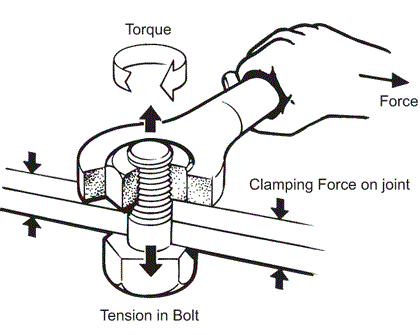## Fastener Torques

Consider you want to join two stainless steel plates together with a 0.190-32" CRES bolt and nut that have a TS = 70,000 psi, friction factor K = 0.2, and a screw tensile stress area of 0.02 in^2. If you want to load the screw with 70% of its tensile strength, what value should you torque (in-lb) the bolt to?Hint
$$Torque=K\times Preload \times Screw\: diameter$$$$$Preload=TS \times TS_{factor}\times Area$$$
First, let's solve for the bolt's preload:
$$Preload=TS \times TS_{factor}\times Area$$$$$Preload=70,000\frac{lb_f}{in^2}\times \frac{70}{100}\times 0.02\:in^2=980\: lb_f$$$
Now we can solve for torque:
$$Torque=K\times Preload \times Screw\: diameter$$$$$Torque=980\: lb_f\times0.2\times 0.19\:in=37\:in\cdot lb_f$$$
$$37\:in\cdot lb_f$$\$